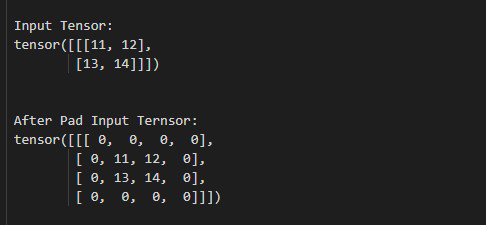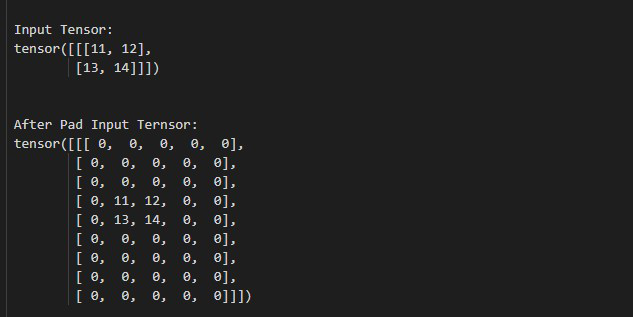Open in App
Not now

# How to Pad the Input Tensor Boundaries with Zero in PyTorch

• Last Updated : 03 May, 2022

In this article, we will discuss how to pad the input tensor boundaries with zero in Python using PyTorch.

This method has accepted the size of padding as input and returns a new tensor with boundaries. The boundaries may be the same or different from all sides (left, right, top, bottom). we can increase the height and width of a padded tensor by using top+bottom and left+right respectively. The below syntax is used to pad the input tensor boundaries with zero.

Parameter:

• pad (int, tuple): This is size of padding. The size of padding is an integer or a tuple.

Return: This method returns a new tensor with boundaries.

Example 1:

In this example, we will see how to pad the input tensor boundaries with zero.

## Python3

 `# Import required library``import` `torch``import` `torch.nn as nn` `# define a tensor``tens ``=` `torch.tensor([[[``11``, ``12``], [``13``, ``14``]]])``print``(``"\n Input Tensor: \n"``, tens)` `# give padding size same for all sides``pad ``=` `nn.ZeroPad2d(``1``)``output ``=` `pad(tens)` `# display result``print``(``"\n After Pad Input Tensor: \n"``, output)`

Output:Example 2:

In this example, we will see how to add unique padding sizes to all sides.

## Python3

 `# Import required library``import` `torch``import` `torch.nn as nn` `# define a tensor``tens ``=` `torch.tensor([[[``11``, ``12``], [``13``, ``14``]]])``print``(``"\n Input Tensor: \n"``, tens)` `# add unique padding sizes to all sides``# (left, right, top, bottom)``pad ``=` `nn.ZeroPad2d((``1``, ``2``, ``3``, ``4``))``output ``=` `pad(tens)` `# display result``print``(``"\n After Pad Input Tensor:\n"``, output)`

Output:My Personal Notes arrow_drop_up